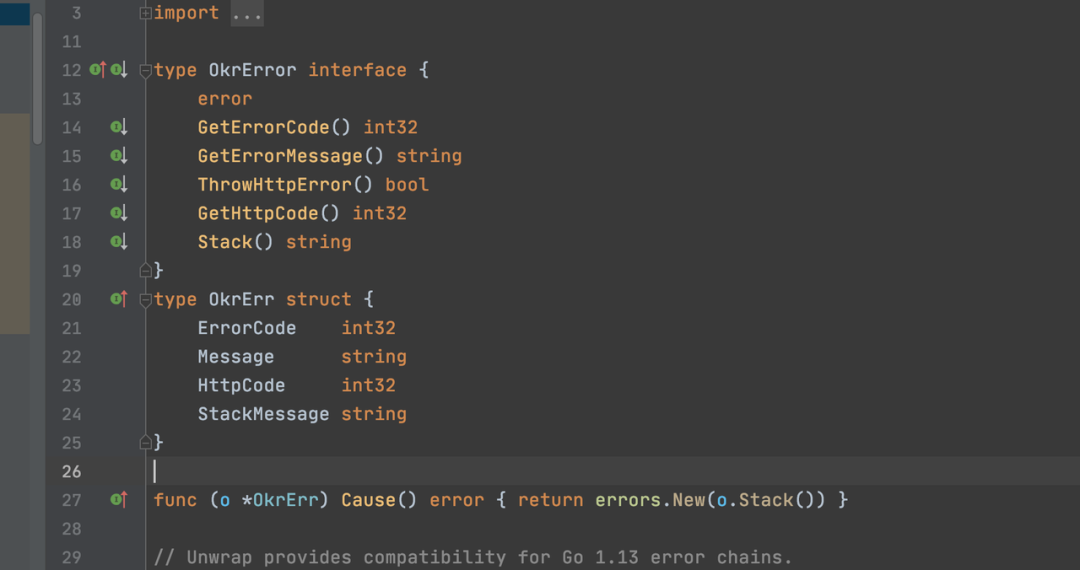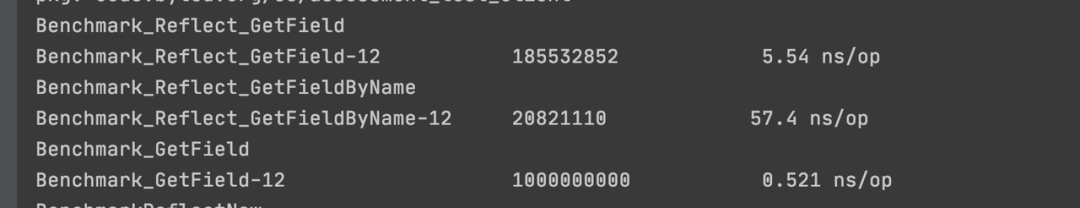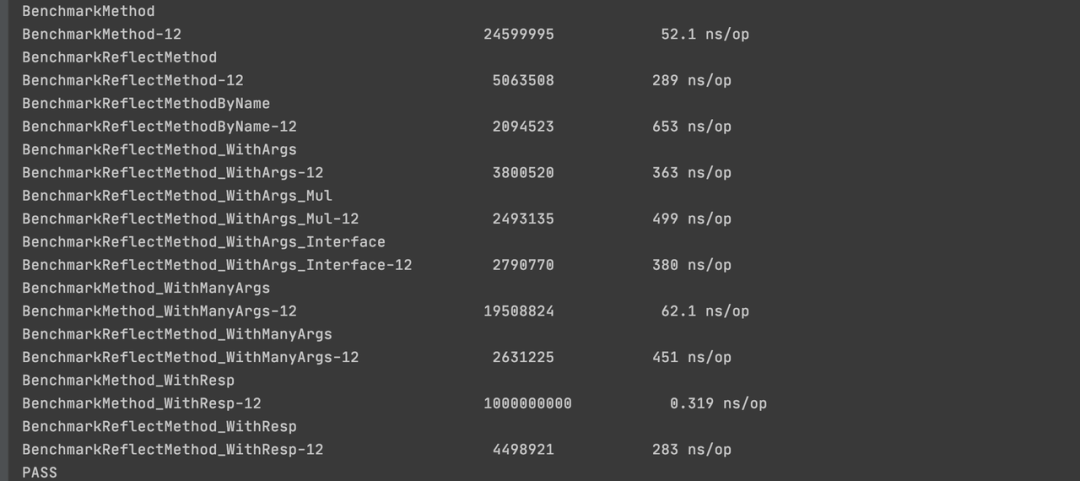Let’s start with a simple piece of code logic to warm up. What do you think should be printed in the following code?

 `````` 1 2 3 4 5 6 7 8 9 10 11 12 13 14 15 16 17 18 19 20 21 22 23 24 25 26 27 28 29 30 31 32 33 34 35 36 37 38 39 40 41 42 43 44 45 46 47 `````` `````` type OKR struct { id int content string } func getOkrDetail(ctx context.Context, okrId int) (*OKR, *okrErr.OkrErr) { return &OKR{id: okrId, content: fmt.Sprint(rand.Int63())}, nil } func getOkrDetailV2(ctx context.Context, okrId int) (*OKR, okrErr.OkrError) { if okrId == 2{ return nil, okrErr.OKRNotFoundError } return &OKR{id: okrId, content: fmt.Sprint(rand.Int63())}, nil } func paperOkrId(ctx context.Context) (int, error){ return 1, nil } func Test001(ctx context.Context) () { var okr *OKR okrId, err := paperOkrId(ctx) if err != nil{ fmt.Println("#### 111 ####") } okr, err = getOkrDetail(ctx, okrId) if err != nil { fmt.Println("#### 222 ####") } okr, err = getOkrDetailV2(ctx, okrId) if err != nil { fmt.Println("#### 333 ####") } okr, err = getOkrDetailV2(ctx, okrId + 1) if err != nil { fmt.Println("#### 444 ####") } fmt.Println("#### 555 ####") fmt.Printf("%v", okr) } func main() { Test001(context.Background()) } ``````## Preface

Before we talk about reflection, let’s take a look at some of the principles of Golang’s type design

• Variables in Golang have two parts (type, value)

• Understanding this will solve the simple problem above

• type includes static type and concrete type. Simply put, static type is the type you see when coding (e.g. int, string) and concrete type is the type seen by the runtime system. The success of type assertion depends on the concrete type of the variable, not the static type.

Next, reflection is the ability to update variables and check their values, call their methods and the intrinsic operations they support at runtime, without knowing the specific types of those variables at compile time. This mechanism is called reflection, and Golang’s base type is static (i.e., variables specifying int, string, etc., whose type is static type) and is determined at the time of variable creation, and reflection is mainly related to Golang’s interface type (whose type is concrete type), and only Only the runtime interface type has reflection.

## Why to use reflection in Golang/what scenarios can (and should) use reflection

When the program runs, we get an interface variable, how should the program know the type of the current variable, and the value of the current variable?

Of course we can have predefined types, but if there is a scenario where we need to write a function that can handle a common type of logic, but there are many input types, or we don’t know what the types of the incoming arguments are, or maybe there is no agreement, or there are many incoming types and they are not represented uniformly. This is where reflection comes in handy, with typical examples such as: `json.Marshal`.

Another example is that sometimes it is necessary to decide which function to call based on certain conditions, such as based on the user’s input. This is where reflection of the function and the function’s arguments is needed to dynamically execute the function during runtime.

### Example scenarios

For example, if we need to perform some kind of operation on a struct (using formatted print instead), there are various ways to achieve this scenario, the simpler way is: switch case

 `````` 1 2 3 4 5 6 7 8 9 10 11 12 13 14 15 16 17 18 19 20 21 22 23 24 `````` `````` func Sprint(x interface{}) string { type stringer interface { String() string } switch x := x.(type) { case stringer: return x.String() case string: return x case int: return strconv.Itoa(x) // int16, uint32... case bool: if x { return "true" } return "false" default: return "wrong parameter type" } } type permissionType int64 ``````

But there is a problem with this simple approach, when adding a scenario, such as slice support, you need to add a branch, which is endless, whenever I need to support a type, even if it is a custom type, which is essentially int64, I still need to add a branch.

## Basic usage of reflection

In Golang, we are provided with two methods, `reflect.ValueOf` and `reflect.TypeOf`, which by their names help us to get the value and type of the object respectively. `Valueof` returns a `Reflect.Value` object, which is a struct, while `Typeof` returns `Reflect.Type` is an interface. We can simply use a combination of these two methods to perform a variety of functions.

 `````` 1 2 3 4 5 6 7 8 9 10 11 12 13 14 15 16 17 18 19 20 21 22 23 24 25 26 27 28 29 30 31 32 33 34 35 36 37 38 39 40 41 42 43 44 45 46 47 48 49 50 51 52 53 54 55 56 57 58 59 60 61 62 63 64 65 66 67 68 69 70 71 72 73 74 75 76 77 78 79 80 81 82 83 84 85 86 87 88 89 90 `````` `````` type GetOkrDetailResp struct { OkrId int64 UInfo *UserInfo ObjList []*ObjInfo } type ObjInfo struct { ObjId int64 Content string } type UserInfo struct { Name string Age int IsLeader bool Salary float64 privateFiled int } // Creating structs using reflection func NewUserInfoByReflect(req interface{})*UserInfo{ if req == nil{ return nil } reqType :=reflect.TypeOf(req) if reqType.Kind() == reflect.Ptr{ reqType = reqType.Elem() } return reflect.New(reqType).Interface().(*UserInfo) } // Modify struct field values func ModifyOkrDetailRespData(req interface{}) { reqValue :=reflect.ValueOf(req).Elem() fmt.Println(reqValue.CanSet()) uType := reqValue.FieldByName("UInfo").Type().Elem() fmt.Println(uType) uInfo := reflect.New(uType) reqValue.FieldByName("UInfo").Set(uInfo) } // Retrieve struct field values and filter by condition func FilterOkrRespData(reqData interface{}, objId int64){ // First get the value of obj slice in req for i := 0 ; i < reflect.ValueOf(reqData).Elem().NumField(); i++{ fieldValue := reflect.ValueOf(reqData).Elem().Field(i) if fieldValue.Kind() != reflect.Slice{ continue } fieldType := fieldValue.Type() // []*ObjInfo sliceType := fieldType.Elem() // *ObjInfo slicePtr := reflect.New(reflect.SliceOf(sliceType)) // Create a pointer to a slice slice := slicePtr.Elem() slice.Set(reflect.MakeSlice(reflect.SliceOf(sliceType), 0, 0)) // Point this pointer to the newly created slice // Filter all structs with objId == current objId for i := 0 ;i < fieldValue.Len(); i++{ if fieldValue.Index(i).Elem().FieldByName("ObjId").Int() != objId { continue } slice = reflect.Append(slice, fieldValue.Index(i)) } // Set the current field of the resp to the filtered slice fieldValue.Set(slice) } } func Test003(){ // Create a new object using reflection var uInfo *UserInfo uInfo = NewUserInfoByReflect(uInfo) uInfo = NewUserInfoByReflect((*UserInfo)(nil)) // Modify the user info field in the resp return value (initialization) reqData1 := new(GetOkrDetailResp) fmt.Println(reqData1.UInfo) ModifyOkrDetailRespData(reqData1) fmt.Println(reqData1.UInfo) // Build request parameters reqData := &GetOkrDetailResp{OkrId: 123} for i := 0; i < 10; i++{ reqData.ObjList = append(reqData.ObjList, &ObjInfo{ObjId: int64(i), Content: fmt.Sprint(i)}) } // Output pre-filter results fmt.Println(reqData) // Filtering operations on respData FilterOkrRespData(reqData, 6) // Output filtered results fmt.Println(reqData) } ``````

We have all heard more or less about the low performance of reflection, using reflection is several times to dozens of times lower than the normal call, I wonder if you have thought about what aspects of reflection performance are low, I first do a simple analysis, through reflection in the acquisition or modification of the value of the content, a few more memory references, a few more times around the corner, certainly not directly call a value to the rapid, this is the fixed performance brought about by reflection Another aspect of performance loss is that when there are more fields of a structure type, you have to iterate through them to get the corresponding content.

The following is a performance analysis based on specific examples of reflection.

### Test reflection structure initialization

 `````` 1 2 3 4 5 6 7 8 9 10 11 12 13 14 15 16 17 18 19 `````` `````` // Testing the reflection performance of structure initialization func Benchmark_Reflect_New(b *testing.B) { var tf *TestReflectField t := reflect.TypeOf(TestReflectField{}) for i := 0; i < b.N; i++ { tf = reflect.New(t).Interface().(*TestReflectField) } _ = tf } // Testing the performance of structure initialization func Benchmark_New(b *testing.B) { var tf *TestReflectField for i := 0; i < b.N; i++ { tf = new(TestReflectField) } _ = tf } ``````

The test results are as follows.As you can see, there is a performance gap between initializing a structure using reflection and creating a new structure directly, but the difference is not significant, less than double the performance loss, which does not seem to be a significant loss in performance and is acceptable.

 `````` 1 2 3 4 5 6 7 8 9 10 11 12 13 14 15 16 17 18 19 20 21 22 23 24 25 26 27 28 29 30 31 32 33 34 35 36 37 38 39 40 41 42 43 44 45 46 47 48 49 50 51 52 53 54 55 56 57 58 59 60 61 62 63 64 65 66 67 68 `````` `````` // --------- ------------ Field Read ----------- ----------- ----------- // Testing the performance of reflective reading of structure field values func Benchmark_Reflect_GetField(b *testing.B) { var tf = new(TestReflectField) var r int64 temp := reflect.ValueOf(tf).Elem() for i := 0; i < b.N; i++ { r = temp.Field(1).Int() } _ = tf _ = r } // Testing the performance of reflective reading of structure field values func Benchmark_Reflect_GetFieldByName(b *testing.B) { var tf = new(TestReflectField) temp := reflect.ValueOf(tf).Elem() var r int64 for i := 0; i < b.N; i++ { r = temp.FieldByName("Age").Int() } _ = tf _ = r } // Test the performance of reading data from structure fields func Benchmark_GetField(b *testing.B) { var tf = new(TestReflectField) tf.Age = 1995 var r int for i := 0; i < b.N; i++ { r = tf.Age } _ = tf _ = r } // --------- ------------ Field Write ----------- ----------- ----------- // Testing the performance of reflective set structure fields func Benchmark_Reflect_Field(b *testing.B) { var tf = new(TestReflectField) temp := reflect.ValueOf(tf).Elem() for i := 0; i < b.N; i++ { temp.Field(1).SetInt(int64(25)) } _ = tf } // Testing the performance of reflective set structure fields func Benchmark_Reflect_FieldByName(b *testing.B) { var tf = new(TestReflectField) temp := reflect.ValueOf(tf).Elem() for i := 0; i < b.N; i++ { temp.FieldByName("Age").SetInt(int64(25)) } _ = tf } // Testing the performance of structure field settings func Benchmark_Field(b *testing.B) { var tf = new(TestReflectField) for i := 0; i < b.N; i++ { tf.Age = i } _ = tf } ``````

The test results are as follows.As you can see above, reading struct fields via reflection takes a hundred times longer than reading them directly. Assigning directly to an instance variable at 0.5 ns each time is about 10 times faster than manipulating the field at the specified location of the instance via reflection. The performance of using `FieldByName("Age")` method is about 10 times lower than using `Field(1)` method. If we look at the code, we will find that `FieldByName` is used to query the position of a field in a structure by iterating through all the fields and then comparing the field names, and then assigning a value by the position, so the performance is much lower than using `Field(index)` is much lower.

Recommendations.

1. If not necessary, try not to use reflection to operate, and when using reflection, evaluate the impact of introducing reflection on performance.
2. Reduce the use of the `FieldByName` method. When you need to use reflection to access a member variable, use the member’s serial number whenever possible. If you only know the name of the member variable, you can get the member’s serial number through `TypeOf()`, `Type.FieldByName()` and `StructField.Index` at startup or before frequent accesses. Note that here it is necessary to use `reflect.Type` instead of `reflect.Value`, which cannot get the name of the field.

### Test struct method calls

 `````` 1 2 3 4 5 6 7 8 9 10 11 12 13 14 15 16 17 18 19 20 21 22 23 24 25 26 27 28 29 30 31 32 33 34 35 36 37 38 39 40 41 42 43 44 45 46 47 48 49 50 51 52 53 54 55 56 57 58 59 60 61 62 63 64 65 66 67 68 69 70 71 72 73 74 75 76 77 78 79 80 81 82 83 84 85 86 `````` `````` // Testing the performance of access methods through structs func BenchmarkMethod(b *testing.B) { t := &TestReflectField{} for i := 0; i < b.N; i++ { t.Func0() } } // Test the performance of calling parameterless methods by serial number reflection func BenchmarkReflectMethod(b *testing.B) { v := reflect.ValueOf(&TestReflectField{}) for i := 0; i < b.N; i++ { v.Method(0).Call(nil) } } // Test the performance of calling parameterless methods by name reflection func BenchmarkReflectMethodByName(b *testing.B) { v := reflect.ValueOf(&TestReflectField{}) for i := 0; i < b.N; i++ { v.MethodByName("Func0").Call(nil) } } // Testing the performance of parameterized method calls via reflection func BenchmarkReflectMethod_WithArgs(b *testing.B) { v := reflect.ValueOf(&TestReflectField{}) for i := 0; i < b.N; i++ { v.Method(1).Call([]reflect.Value{reflect.ValueOf(i)}) } } // Test the performance of the method of calling structure parameters by reflection func BenchmarkReflectMethod_WithArgs_Mul(b *testing.B) { v := reflect.ValueOf(&TestReflectField{}) for i := 0; i < b.N; i++ { v.Method(2).Call([]reflect.Value{reflect.ValueOf(TestReflectField{})}) } } // Test the performance of the interface parameter method invocation by reflection func BenchmarkReflectMethod_WithArgs_Interface(b *testing.B) { v := reflect.ValueOf(&TestReflectField{}) for i := 0; i < b.N; i++ { var tf TestInterface = &TestReflectField{} v.Method(3).Call([]reflect.Value{reflect.ValueOf(tf)}) } } // Testing the performance of calling multi-parameter methods func BenchmarkMethod_WithManyArgs(b *testing.B) { s := &TestReflectField{} for i := 0; i < b.N; i++ { s.Func4(i, i, i, i, i, i) } } // Testing the performance of multiparameter method calls via reflection func BenchmarkReflectMethod_WithManyArgs(b *testing.B) { v := reflect.ValueOf(&TestReflectField{}) va := make([]reflect.Value, 0) for i := 1; i <= 6; i++ { va = append(va, reflect.ValueOf(i)) } for i := 0; i < b.N; i++ { v.Method(4).Call(va) } } // Testing the performance of calling methods with return values func BenchmarkMethod_WithResp(b *testing.B) { s := &TestReflectField{} for i := 0; i < b.N; i++ { _ = s.Func5() } } // Testing the performance of methods called by reflection with return values func BenchmarkReflectMethod_WithResp(b *testing.B) { v := reflect.ValueOf(&TestReflectField{}) for i := 0; i < b.N; i++ { _ = v.Method(5).Call(nil).Int() } } ``````The results of this test are the same as the analysis above.

### Pros and Cons

Pros.

1. reflection improves program flexibility and extensibility, reduces coupling, and improves self-adaptability.
2. Rational use of reflection can reduce duplicate code

1. The code associated with reflection is often difficult to read. In software engineering, code readability is also a very important metric.
2. Go, being a static language, is coded in such a way that the compiler can find some type errors in advance, but it can’t do anything about reflection code. So the code containing reflection-related code will probably run for a long time before an error occurs. This time the program will often just panic, which may cause serious consequences.
3. reflection still has a significant impact on performance, running one to two orders of magnitude slower than normal code. Therefore, for a project in a critical position in the running efficiency of the code, try to avoid using reflection features.

## A simple application of reflection in okr

 `````` 1 2 3 4 5 6 7 8 9 10 11 12 13 14 15 16 17 18 19 20 21 22 23 24 25 26 27 28 29 30 31 32 33 34 35 36 37 38 39 `````` `````` func OkrBaseMW(next endpoint.EndPoint) endpoint.EndPoint { return func(ctx context.Context, req interface{}) (resp interface{}, err error) { if req == nil { return next(ctx, req) } requestValue := reflect.ValueOf(req) // If req is a pointer, then convert to a non-pointer value if requestValue.Type().Kind() == reflect.Ptr { requestValue = requestValue.Elem() } // If the value of req is not a struct, it is not injected if requestValue.Type().Kind() != reflect.Struct { return next(ctx, req) } if requestValue.IsValid() { okrBaseValue := requestValue.FieldByName("OkrBase") if okrBaseValue.IsValid() && okrBaseValue.Type().Kind() == reflect.Ptr { okrBase, ok := okrBaseValue.Interface().(*okrx.OkrBase) if ok { ctx = contextWithUserInfo(ctx, okrBase) ctx = contextWithLocaleInfo(ctx, okrBase) ctx = contextWithUserAgent(ctx, okrBase) ctx = contextWithCsrfToken(ctx, okrBase) ctx = contextWithReferer(ctx, okrBase) ctx = contextWithXForwardedFor(ctx, okrBase) ctx = contextWithHost(ctx, okrBase) ctx = contextWithURI(ctx, okrBase) ctx = contextWithSession(ctx, okrBase) } } } return next(ctx, req) } } ``````

## Summary

Using reflection will definitely lead to performance degradation, but reflection is a powerful tool that can solve many of our usual problems, such as database mapping, data serialization, and code generation scenarios. When using reflection, we need to avoid some low performance operations, such as using `FieldByName()` and `MethodByName()` methods. If we have to use these methods, we can improve the performance of using reflection by getting the corresponding field number by field name or method name in advance, and then using the higher performance reflection operations.

## Reference

• `https://mp.weixin.qq.com/s?__biz=MzI1MzYzMjE0MQ==&mid=2247497793&idx=1&sn=00456bed305439b7f50838eae8353056`